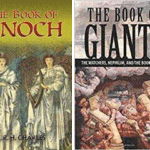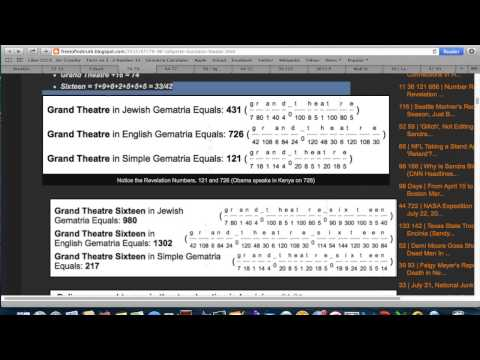Through the Scorned Eyes of Simplicio….About That Creepy New Statue Atop the New York City CourthouseThe Contrived Calvinist vs Arminianist Division and the Gospel of Jesus ChristIn The Beginning Was The Word | The Resurrection of the Hebrew Language and Suppression of the TruthSouthwest Airlines. My Christmas Story 2022Is Christianity Just Another Religion?Unidentified Floating Objects…The Book of Enoch is a Dangerous Demonic Snare# Louisiana Theater Shooting Hoax- The Aurora, CO Connection & “Why Trainwreck?”RedsilverjJul 23, 2015 by Zachary K Hubbard

33 74 79 96 122 | Lafayette, Louisiana Grand Theater 16 Shooting Initial Reporting (Looks Hoax-a-Licious)

Shots = 1+8+6+2+1 = 18/27/36
Fired = 6+9+9+5+4 33
At = 1+2 = 3
Theater = 2+8+5+1+2+5+9 = 32
Shots Fired at Theater = 86/95/104
Symbol = 86
Triangle = 86
Pyramid = 86

Lafayette = 3+1+6+1+7+5+2+2+5 = 32
Louisiana = 3+6+3+9+1+9+1+5+1 = 38/47
Lafayette, Lousiana = 70/79
Murder = 79

The theatre was ‘Grand Theatre 16 (Sixteen)’.
Grand = 7+9+1+5+4 = 26
Theatre = 2+8+5+1+2+9+5 = 32
Grand Theatre = 58
58-Year Old Shooter
Grand Theatre +16 = 74
Sixteen = 1+9+6+2+5+5+5 = 33/42

Notice the Revelation Numbers, 121 and 726 (Obama speaks in Kenya on 726)

Police = 7+6+3+9+3+5 = 33
Chief = 3+8+9+5+6 = 31
Jim = 1+9+4 = 14
Craft = 3+9+1+6+2 = 21
Police Chief Jim Craft = 99

Todd = 2+6+4+4 = 16
Moffatt = 4+6+6+6+1+2+2 = 27
Todd Moffatt = 43

Todd is manager of Mellow Mushroom Pizza.
Mellow = 4+5+3+3+6+5 = 26
Mushroom = 4+3+1+8+9+6+6+4 = 41/50
Pizza = 7+9+8+8+1 = 33
Mellow Mushroom Pizza = 100/109

22 Books in Revelation
The Governor’s name has familiar Gematria.
Bobby = 2+6+2+2+7 = 19
Jindal = 1+9+5+4+1+3 = 23
Bobby Jindal = 42

Update by  Obama 793

The Suspect Named as “John Russell Houser” = 17 letter Characters.

“John Russell Houser”  Reduced English = 77

# The Number “17”

MH17, 17 Shot on 17th 7th 2014

17 shot at Charlie Hebdo,

Benjamin Netanyahu = 17 Characters, re elected on 17th March.

17 leaves in each of the two olive branches on the Mossad Crest.

Follow